Using JMP > Create Formulas in JMP > Refer to Data Table Values in Formulas
Publication date: 05/24/2021

# Refer to Data Table Values in Formulas

You can create a formula that refers to values found in other parts of the data table, such as other columns and table variables.

When a formula uses values in other columns, the values in the column with that formula are dependent on the values in those other columns. Whenever a column that the formula refers to changes, the dependent column also changes. If you delete the referenced column, empty terms appear in the column containing the formula.

If you create a formula that refers to values found in table variables, those table variables must already exist in the data table. Table variables are character strings that are available to the entire table. Their names are displayed in the table panel at the left of the data table. See Use Data Table Variables.

Tip: To create a table variable from a value in the Formula Editor, right-click the value and select Create Table Variable from Value.

The following example shows how to re-create a formula column in the Companies.jmp sample data table.

1. Select Help > Sample Data Library and open Companies.jmp.

2. Right-click the last empty column and select New Columns.

The Column Info window appears.

3. Type “Profit by Sales” next to Column Name.

You are re-creating the formula found in the existing %profit/sales column.

4. In the Column Properties list, select Formula.

The Formula Editor opens. The blue outline around the box in the editing window indicates that the box is selected.

5. With the box selected, select Profits (\$M) from the Columns list.

Figure 7.3 Adding the Profits (\$M) Column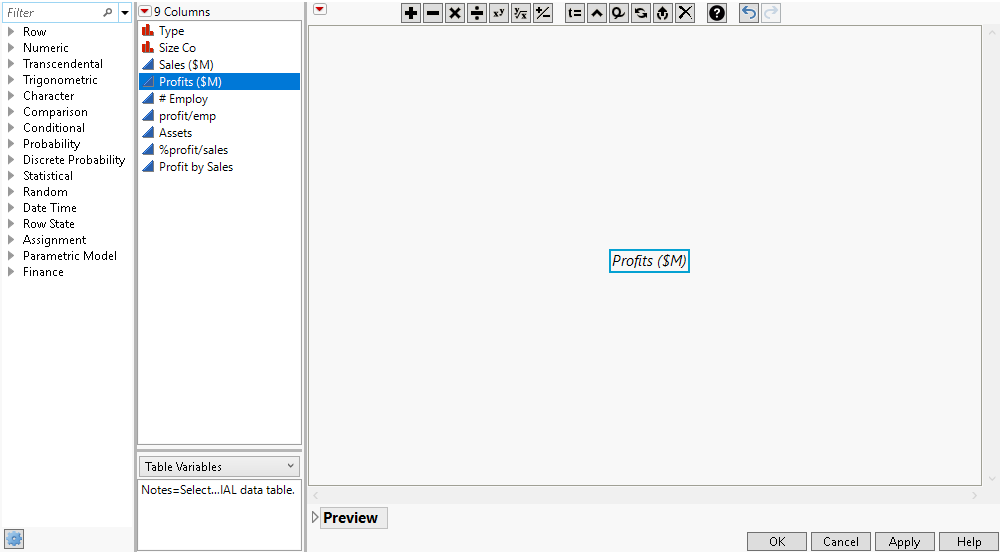6. With the box selected, click the Divide button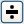.

Figure 7.4 Adding the Division Symbol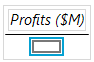7. With the box selected as shown, select Sales (\$M) from the Columns list.

Figure 7.5 Adding the Sales (\$M) Column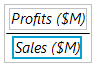8. Select the outer box in the formula editing area by clicking it.

Figure 7.6 Selecting the Formula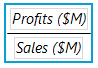9. Click the Multiply button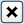.

Figure 7.7 Adding the Multiplication Symbol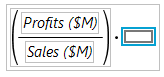10. With the new box selected, type “100” and press Enter.

Figure 7.8 Multiplying the Formula by 100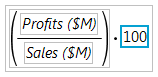11. Click OK.

The column is filled with the calculated numbers.

12. Right-click the last column and select Column Info.

13. In the Column Info window, select Fixed Dec from the Format list, type “10” next to Width, and type “2” next to Dec.

The column width and number of decimal places are specified.

14. Click OK.

The new formula column matches the %profit/sales formula column.

Tips:

Pressing Alt (Option on macOS) and clicking a column replaces both empty and non-empty selected fields with that column.

The right-click menu for the Columns list has two replacement options: Replace all occurrences of selected subexpression replaces all occurrences of the selected expression with the currently selected column. Replace the selected subexpression with columns replaces the selected expression with multiple columns for functions that support it (for example, Sum or Plus).

Define your own functions and transform columns by writing a JSL script. See Create Custom Functions, Transforms, and Formats in the Scripting Guide.